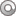• Price: \$50.00 Quantity:
Doors will open at 6:00pm, followed by introductions. Class will start at 6:30 and typically ends by 8pm.
• Price: \$50.00 Quantity:
Doors will open at 6:00pm, followed by introductions. Class will start at 6:30 and typically ends by 8pm.
• Price: \$50.00 Quantity:
Doors will open at 6:00pm, followed by introductions. Class will start at 6:30 and typically ends by 8pm.
• Price: \$50.00 Quantity:
Doors will open at 6:00pm, followed by introductions. Class will start at 6:30 and typically ends by 8pm.
• Price: \$50.00 Quantity:
Doors will open at 6:00pm, followed by introductions. Class will start at 6:30 and typically ends by 8pm.
• Price: \$50.00 Quantity:
Doors will open at 6:00pm, followed by introductions. Class will start at 6:30 and typically ends by 8pm.
• Price: \$50.00 Quantity:
Doors will open at 6:00pm, followed by introductions. Class will start at 6:30 and typically ends by 8pm.
• Price: \$50.00 Quantity:
Doors will open at 6:00pm, followed by introductions. Class will start at 6:30 and typically ends by 8pm.
When you leave your parking spot do you see spots underneath??
• List any leaks, sounds, visual issues you are noticing with your car.
•• \$0.00